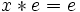# Magma

Jump to: navigation, search

## Definition

A set equipped with a binary operation is termed a magma.

Magmas are also sometimes termed groupoids, but the term groupoid has another more common use.

## Relation with other structures

### Additional structures and conditions

The following are the common choices of additional conditions usually imposed on the binary operation of a magma:

• The existence of a neutral element, i.e., an identity element for the binary operation. For a binary operation$*$, a left neutral element is an element$e$ such that$e * x = x$ for all$x$ and a right neutral element is an element$e$ such that$x * e = e$ for all$x$. A (two-sided) neutral element is an element that is both left and right neutral.
• The associativity condition, which states that$a * (b * c) = (a * b) * c$ for all$a,b,c$ in the underlying set of the magma. There are many weaker versions of associativity, which require only certain kinds of expressions to associate.
• The commutativity condition, which states that$a * b = b * a$ for all$a,b$ in the underlying set of the magma. There are weaker versions of commutativity, which requre only certain kinds of expressions to commute.
• The existence of a nil element, i.e., a zero element for the binary operation.$n$ is a left nil for$*$ if$n * x = n$ for all$x$, and a right nil for$*$ if$x * n = n$ for all$x$.
• The existence of inverse elements with respect to a two-sided neutral element. A left inverse for$a$ with respect to$e$ is a$b$ such that$b * a = e$, and a right inverse is a$b$ such that$a * b = e$.
• Cancellation. An element$a$ is left-cancellative if$a * b = a * c \implies b = c$ and right-cancellative if$b * a = c * a \implies b = c$.
• Existence of solutions to left/right quotient equations: Whether an equation of the form$a * x = b$ can be solved, for given$a,b$. Similarly, whether$y * a = b$ can be solved for given$a,b$.

### Terminology for magmas with these additional conditions

A Yes means that the structure/condition is always true for that type of magma while a No means that it is not necessarily true. The collection of Yeses in any row completely defines the type of magma being considered.

Name Two-sided neutral element Associativity Commutativity Inverses Cancellation Existence of quotients
Magma No No No No No No
Unital magma Yes No No No No No
Cancellative magma No No No No Yes No
Semigroup No Yes No No No No
Cancellative semigroup No Yes No No Yes No
Monoid Yes Yes No No No No
Quasigroup No No No No Yes Yes
Algebra loop Yes No No Yes Yes Yes
Group Yes Yes No Yes Yes Yes
Abelian group Yes Yes Yes Yes Yes Yes